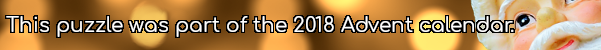mscroggs.co.uk
mscroggs.co.uksubscribe

# Puzzles

## 10 DecemberFor all values of $$x$$, the function $$f(x)=ax+b$$ satisfies
$$8x-8-x^2\leqslant f(x)\leqslant x^2.$$
What is $$f(65)$$?
Edit: The left-hand quadratic originally said $$8-8x-x^2$$. This was a typo and has now been corrected.

## 10 DecemberThe equation $$x^2+1512x+414720=0$$ has two integer solutions.
Today's number is the number of (positive or negative) integers $$b$$ such that $$x^2+bx+414720=0$$ has two integer solutions.

$$(x^2-7x+11)^{(x^2-11x+30)}=1.$$